# JP2016219995A - Radio communication system, base station, radio terminal, and base station processing method - Google Patents

## Info

Publication number
JP2016219995A
JP2016219995A JP2015102159A JP2015102159A JP2016219995A JP 2016219995 A JP2016219995 A JP 2016219995A JP 2015102159 A JP2015102159 A JP 2015102159A JP 2015102159 A JP2015102159 A JP 2015102159A JP 2016219995 A JP2016219995 A JP 2016219995A
Authority
JP
Japan
Prior art keywords
plurality
base station
wireless
cell
transmission power
Prior art date
Legal status (The legal status is an assumption and is not a legal conclusion. Google has not performed a legal analysis and makes no representation as to the accuracy of the status listed.)
Pending
Application number
JP2015102159A
Other languages
Japanese (ja)
Inventor

Takashi Seyama

Original Assignee

Fujitsu Ltd
Priority date (The priority date is an assumption and is not a legal conclusion. Google has not performed a legal analysis and makes no representation as to the accuracy of the date listed.)
Filing date
Publication date
Application filed by 富士通株式会社, Fujitsu Ltd filed Critical 富士通株式会社
Priority to JP2015102159A priority Critical patent/JP2016219995A/en
Publication of JP2016219995A publication Critical patent/JP2016219995A/en
Pending legal-status Critical Current

• 238000004891 communication Methods 0.000 title claims abstract description 68
• 238000003672 processing method Methods 0.000 title claims description 7
• 230000005540 biological transmission Effects 0.000 claims abstract description 153
• 238000000034 methods Methods 0.000 claims description 38
• 239000003138 indicators Substances 0.000 claims 3
• 239000000203 mixtures Substances 0.000 description 12
• 230000000051 modifying Effects 0.000 description 10
• 238000005516 engineering processes Methods 0.000 description 4
• 239000000835 fibers Substances 0.000 description 1

## Images

•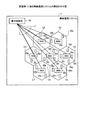•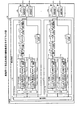•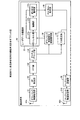•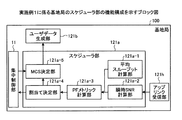•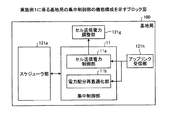•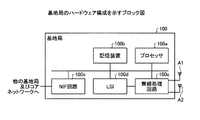•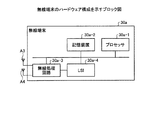•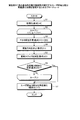•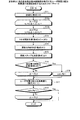•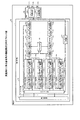•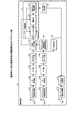•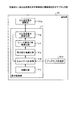•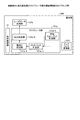•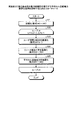•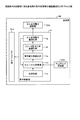•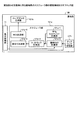•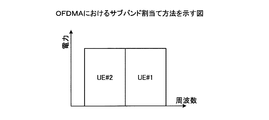•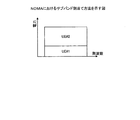•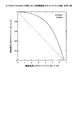## Classifications

• HELECTRICITY
• H04ELECTRIC COMMUNICATION TECHNIQUE
• H04BTRANSMISSION
• H04B7/02Diversity systems; Multi-antenna system, i.e. transmission or reception using multiple antennas
• H04B7/04Diversity systems; Multi-antenna system, i.e. transmission or reception using multiple antennas using two or more spaced independent antennas
• H04B7/0413MIMO systems
• HELECTRICITY
• H04ELECTRIC COMMUNICATION TECHNIQUE
• H04BTRANSMISSION
• H04B7/02Diversity systems; Multi-antenna system, i.e. transmission or reception using multiple antennas
• H04B7/022Site diversity; Macro-diversity
• HELECTRICITY
• H04ELECTRIC COMMUNICATION TECHNIQUE
• H04WWIRELESS COMMUNICATION NETWORKS
• H04W52/00Power management, e.g. TPC [Transmission Power Control], power saving or power classes
• H04W52/04TPC
• H04W52/18TPC being performed according to specific parameters
• H04W52/24TPC being performed according to specific parameters using SIR [Signal to Interference Ratio] or other wireless path parameters
• H04W52/241TPC being performed according to specific parameters using SIR [Signal to Interference Ratio] or other wireless path parameters taking into account channel quality metrics, e.g. SIR, SNR, CIR, Eb/lo
• HELECTRICITY
• H04ELECTRIC COMMUNICATION TECHNIQUE
• H04WWIRELESS COMMUNICATION NETWORKS
• H04W52/00Power management, e.g. TPC [Transmission Power Control], power saving or power classes
• H04W52/04TPC
• H04W52/18TPC being performed according to specific parameters
• H04W52/24TPC being performed according to specific parameters using SIR [Signal to Interference Ratio] or other wireless path parameters
• H04W52/243TPC being performed according to specific parameters using SIR [Signal to Interference Ratio] or other wireless path parameters taking into account interferences
• HELECTRICITY
• H04ELECTRIC COMMUNICATION TECHNIQUE
• H04WWIRELESS COMMUNICATION NETWORKS
• H04W52/00Power management, e.g. TPC [Transmission Power Control], power saving or power classes
• H04W52/04TPC
• H04W52/30TPC using constraints in the total amount of available transmission power
• H04W52/34TPC management, i.e. sharing limited amount of power among users or channels or data types, e.g. cell loading
• HELECTRICITY
• H04ELECTRIC COMMUNICATION TECHNIQUE
• H04WWIRELESS COMMUNICATION NETWORKS
• H04W52/00Power management, e.g. TPC [Transmission Power Control], power saving or power classes
• H04W52/04TPC
• H04W52/38TPC being performed in particular situations
• H04W52/386TPC being performed in particular situations centralized, e.g. when the radio network controller or equivalent takes part in the power control
• HELECTRICITY
• H04ELECTRIC COMMUNICATION TECHNIQUE
• H04WWIRELESS COMMUNICATION NETWORKS
• H04W52/00Power management, e.g. TPC [Transmission Power Control], power saving or power classes
• H04W52/04TPC
• H04W52/06TPC algorithms

## Abstract

An object of the present invention is to improve the fairness of communication opportunities of wireless terminals in a plurality of cells. A base station is capable of wireless communication with a plurality of wireless terminals. Base station 100 includes a plurality of RRHs 20a to 20g and a cell transmission power control unit. The plurality of RRHs 20a to 20g form a plurality of cells. The cell transmission power control unit transmits the transmission power of each cell so that the value of the index indicating the degree of fairness of the communication opportunity of the wireless terminal in each of the plurality of cells is the maximum for the plurality of cells. To decide. The plurality of RRHs 20a to 20g transmit signals to the plurality of wireless terminals by the cell transmission power determined by the cell transmission power control unit in the cell where each wireless terminal is located. [Selection] Figure 2

## Description

The present invention relates to a radio communication system, a base station, a radio terminal, and a base station processing method.

Conventionally, OFDMA (Orthogonal Frequency Division Multiple Access) is used in the downlink of 3GPP (Long Term Evolution) 3GPP (Long Term Evolution), which is a representative of the fourth generation mobile communication system. OFDMA is a method of orthogonal multiple access (OMA). FIG. 17A is a diagram illustrating a subband allocation method in OFDMA. In OFDMA, as shown in FIG. 17A, when the base station schedules data to a plurality of wireless terminals (UE # 1, UE # 2), mutual interference does not occur between the wireless terminals in the same time zone. , Orthogonal subbands are assigned.

On the other hand, in the fifth generation mobile communication system, a non-orthogonal multiple access (NOMA) system in which a base station allocates non-orthogonal resources that interfere with each other to a plurality of wireless terminals has been studied. Yes. FIG. 17B is a diagram illustrating a subband allocation method in NOMA. As shown in FIG. 17B, in NOMA, a base station allocates the same subband to two radio terminals (UE # 1, UE # 2) with a predetermined power distribution. For example, there is a NOMA system having a SIC (Successive Interference Canceller) function that demodulates and decodes a signal for a local terminal after canceling a signal for another terminal that causes interference due to a plurality of wireless terminals being simultaneously multiplexed on the same resource. Proposed.

In NOMA, the base station selects two wireless terminals (users), that is, a wireless terminal U1 that is close to the local station and has a high signal-to-noise ratio (SNR), and a wireless terminal U2 that is far from the local station and has a low SNR. To do. Since the SNR of the radio terminal U2 is lower than the SNR of the radio terminal U1, the modulation and coding scheme (MCS) of the signal for the radio terminal U2 is set low. For this reason, the radio | wireless terminal U1 can make the demodulation and decoding of the signal for radio | wireless terminal U2 succeed with high probability. Therefore, the radio terminal U1 can reduce the influence of interference from the signal for the radio terminal U2 by canceling the signal for the radio terminal U2 that has been successfully demodulated and decoded from the received signal.

On the other hand, in the wireless terminal U2, the influence of interference from the signal for the wireless terminal U1 is large. However, as described above, the SNR of the radio terminal U2 is low and the influence of other interference noise is large, so the influence of interference from the signal for the radio terminal U1 is relatively small. FIG. 18 is a diagram illustrating a difference in capacity of the wireless terminals U1 and U2 between OFDMA and NOMA. In FIG. 18, the capacity (unit: bit / s / Hz) of the wireless terminal U1 is defined on the x axis, and the capacity (unit: bit / s / Hz) of the wireless terminal U2 is defined on the y axis. In FIG. 18, the SNR of the radio terminal U1 is assumed to be 20 dB, and the SNR of the radio terminal U2 is assumed to be 0 dB. As shown in FIG. 18, since NOMA indicated by a solid line is always located in the upper right region than OFDMA indicated by a broken line, the capacity of wireless terminals U1 and U2 is always higher for NOMA than for OFDMA. It can be seen that the system performance is improved.

A. Benjebbour, Y. Saito, Y. Kishiyama, A. Li, A. Harada, and T. Nakamura, "Concept and practical considerations of non-orthogonal multiple access (NOMA) for future radio access," ISPACS 2013, Nov. 2013.

Here, as one of the radio resource scheduling in which the base station decides how much resources are allocated to which radio terminal among the radio terminals belonging to the own station cell, the fairness between the radio terminals is set. There is PF (Proportional Fair) scheduling in consideration. Even in a system to which NOMA is applied, it is possible to maximize the PF utility (logarithm of the average throughput of each wireless terminal), which is the fairness index, by PF scheduling. However, in the PF scheduling closed in the cell, the PF utility in the own cell is maximized, but the PF utility of all cells including other cells is not always maximized.

The disclosed technology has been made in view of the above, and in a plurality of cells, the wireless communication system, the base station, the wireless terminal, and the processing of the base station that can improve the fairness of the communication opportunity of the wireless terminal It aims to provide a method.

In one aspect, a wireless communication system includes a base station and a plurality of wireless terminals capable of wireless communication with the base station. The base station includes a plurality of wireless devices and a power determination unit. The plurality of wireless devices form a plurality of cells. The power determination unit determines the transmission power of each cell so that the value of the index indicating the degree of fairness of the communication opportunity of the wireless terminal in each of the plurality of cells is maximized for the plurality of cells. . The plurality of wireless terminals have a communication unit. The communication unit receives a signal from each of the plurality of wireless devices by the transmission power of the cell determined by the power determination unit in the cell where each wireless terminal is located.

According to one aspect of the wireless communication system, the base station, the wireless terminal, and the processing method of the base station disclosed in the present application, it is possible to improve the fairness of the communication opportunity of the wireless terminal in a plurality of cells.

FIG. 1 is a diagram illustrating the configuration of the wireless communication system according to the first embodiment. FIG. 2 is a block diagram illustrating a functional configuration of the base station according to the first embodiment. FIG. 3 is a block diagram illustrating a functional configuration of the wireless terminal according to the first embodiment. FIG. 4 is a block diagram illustrating a functional configuration of the scheduler unit of the base station according to the first embodiment. FIG. 5 is a block diagram illustrating a functional configuration of the centralized control unit of the base station according to the first embodiment. FIG. 6 is a block diagram illustrating a hardware configuration of the base station. FIG. 7 is a block diagram illustrating a hardware configuration of the wireless terminal. FIG. 8 is a flowchart for explaining the inter-user power distribution reoptimization process executed by the central control unit of the base station according to the first embodiment. FIG. 9 is a flowchart for explaining the inter-user power distribution reoptimization process executed by the central control unit of the base station according to the first modification. FIG. 10 is a block diagram illustrating a functional configuration of the base station according to the second embodiment. FIG. 11 is a block diagram illustrating a functional configuration of a wireless terminal according to the second embodiment. FIG. 12 is a block diagram illustrating a functional configuration of the centralized control unit of the base station according to the third embodiment. FIG. 13 is a block diagram illustrating a functional configuration of the scheduler unit of the base station according to the third embodiment. FIG. 14 is a flowchart for explaining the average cell transmission power optimization process executed by the central control unit of the base station according to the third embodiment. FIG. 15 is a block diagram illustrating a functional configuration of a central control unit of a base station according to another aspect of the third embodiment. FIG. 16 is a block diagram illustrating a functional configuration of the scheduler unit of the base station according to another aspect of the third embodiment. FIG. 17A is a diagram illustrating a subband allocation method in OFDMA. FIG. 17B is a diagram illustrating a subband allocation method in NOMA. FIG. 18 is a diagram illustrating a difference in capacity of a wireless terminal between OFDMA and NOMA.

Hereinafter, embodiments of a wireless communication system, a base station, a wireless terminal, and a processing method of the base station disclosed in the present application will be described in detail with reference to the drawings. Note that the present embodiment does not limit the radio communication system, base station, radio terminal, and base station processing method disclosed in the present application.

Hereinafter, a configuration of a wireless communication system according to an embodiment disclosed in the present application will be described. FIG. 1 is a diagram illustrating a configuration of a wireless communication system 1 according to the first embodiment. As shown in FIG. 1, the radio communication system 1 includes one central control station 10, a plurality of RRHs (Remote Radio Heads) 20a to 20g, and a plurality of radio terminals 30a to 30q. The central control station 10 is connected to a plurality of RRHs 20a to 20g via the optical fiber F. The RRHs 20a to 20g form cells C1 to C7, respectively, and perform wireless communication with the wireless terminals 30a to 30q located in each of the cells C1 to C7.

FIG. 2 is a block diagram illustrating a functional configuration of the base station 100 according to the first embodiment. As illustrated in FIG. 2, the base station 100 includes a central control station 10 and RRHs 20a to 20g. Furthermore, the centralized control station 10 includes a centralized control unit 11 that comprehensively controls each unit of the base station 100, and baseband units 121 to 127 that perform baseband processing of the cells C1 to C7.

As will be described in detail later, the scheduler unit 121a performs radio terminal (a plurality of radio terminals in the case of NOMA) to which resources are allocated at a predetermined time, the power of each radio terminal, and the modulation coding rate ( MCS). In addition, the scheduler unit 121a notifies the central control unit 11 of the allocation target wireless terminal and the power of each wireless terminal. The central control unit 11 determines the transmission power of the cell C1 based on the notification result.

The user data generation unit 121b generates user data for the wireless terminal user instructed from the scheduler unit 121a by the data generation unit 121b-1. The error correction coding unit 121b-2 performs error correction coding of user data at the coding rate for the wireless terminal user specified by the scheduler unit 121a. The modulation unit 121b-3 modulates user data by the modulation method instructed by the scheduler unit 121a. The user power adjustment unit 121b-4 adjusts the power allocated to the user data transmitted to the user to the power instructed by the scheduler unit 121a.

The non-orthogonal multiplexing unit 121c multiplexes data after power adjustment of a plurality of wireless terminals instructed by the scheduler unit 121a. A downlink control signal generation unit (not shown) generates control signals such as MCS and power of each user radio terminal. The channel multiplexing unit 121d multiplexes user data and control signals that have been subjected to non-orthogonal multiplexing. An IFFT (Inverse Fast Fourier Transform) unit 121e performs inverse fast Fourier transform on the multiplexed user data and control signal to convert them into effective symbols. A CP (Cyclic Prefix) adding unit 121f adds a CP to a valid symbol to generate an OFDM symbol. The cell transmission power adjustment unit 121g controls the power of the transmission signal output from the baseband unit 121 to be the power notified from the central control unit 11, thereby transmitting each cell (for example, the cell C1). Control power. The transmission power adjustment process may be performed on the RRH 20a side instead of the central control station 10 side.

The RRH 20a converts the digital transmission signal output from the baseband unit 121 into an analog signal by the downlink radio processing unit 20a-1. Then, the RRH 20a uses the downlink radio processing unit 20a-1 to up-convert the transmission signal converted into the analog signal to the radio frequency of the OFDM symbol, and transmits it from the transmission antenna A1a to the radio terminal (for example, the radio terminal 30a). Send to.

The configuration of the baseband unit 121 and the RRH 20a has been described above representatively, but the configurations of the other baseband units 122 to 127 and the RRHs 20b to 20g are the same as the configurations of the baseband unit 121 and the RRH 20a. Accordingly, common constituent elements are denoted by the same reference numerals at the end, and illustration and detailed description thereof are omitted.

FIG. 3 is a block diagram illustrating a functional configuration of the wireless terminal 30 according to the first embodiment. As shown in FIG. 3, the downlink radio processing unit 31 down-converts the radio frequency signal received from the reception antenna A8a into a baseband signal. Then, the downlink radio processing unit 31 converts the down-converted analog reception signal into a digital signal. The CP removal unit 32 removes the CP from the received OFDM symbol and obtains an effective symbol. An FFT (Fast Fourier Transform) unit 33 performs a fast Fourier transform on the effective symbol and converts it to a frequency domain signal. The channel demapping unit 34 extracts a control signal from the signal converted into the frequency domain signal, and outputs the control signal to the CSI estimation unit 35 and the downlink control signal demodulation / decoding unit 36. Although details will be described later, the CSI estimation unit 35 estimates channel quality information (CSI). The downlink control signal demodulation / decoding unit 36 demodulates the received control signal, and acquires information on MCS and power of each user included in the control signal.

The user data demodulation unit 37 includes a cancel unit 37a, a demodulation unit 37b, and an error correction decoding unit 37c. The cancel unit 37a cancels non-orthogonal multiplexed users. When only the own radio terminal 30 is an assignment target, that is, when there is no non-orthogonal multiplex user other than the own terminal, the user data demodulator 37 skips the processing of the cancel unit 37a. Then, the user data demodulator 37 obtains user data for the terminal itself through the demodulation process by the demodulator 37b and the error correction decoding process by the error correction decoder 37c. On the other hand, when there are non-orthogonal multiplex users other than the own terminal, the user data demodulator 37 performs demodulation processing and error correction of other non-orthogonal multiplex users whose MCS is lower than the MCS of the own terminal and has the lowest MCS. Perform decryption processing. When the error correction decoding process is successful, the user data demodulator 37 cancels the corresponding user data from the received signal by the cancel unit 37a.

Hereinafter, the user data demodulating unit 37 repeatedly executes the above-described demodulation, error correction decoding, and cancellation processes in the order of MCS lower for non-orthogonal multiplexed users whose MCS is lower than that of its own terminal. As a result, when the user data processing of all non-orthogonal multiplexed users whose MCS is lower than the MCS of the own terminal is completed, the user data demodulating unit 37 executes the demodulation processing and error correction decoding processing of the user data of the own terminal. .

The Ack / Nack generation unit 38 generates an Ack signal when the error correction decoding of the user data of the terminal itself is successful, and generates a Nack signal when it fails. The uplink transmission unit 39 performs error correction coding and modulation on the uplink user data, the Ack / Nack signal, and the channel quality information (CSI). Uplink radio processing section 310 converts an encoded and modulated digital transmission signal or the like into an analog signal, up-converts the converted signal to a radio frequency, and transmits the signal from transmission antenna A8b to base station 100. To do.

Here, the processing of the CSI estimation unit 35 will be described in detail. Assuming that the number of transmitting antennas of each of the cells C1 to C7 is 1, and the number of receiving antennas of the wireless terminals 30a to 30q is N rx , the received signal of the kth user (wireless terminal) connected to the i th cell is It is represented by the following mathematical formula (1). Note that i and k are natural numbers.

However, y i, k is a received signal vector of N rx × 1, and h i, k, j is N rx × between the k-th user connected to the i-th cell and the j-th cell. 1 is a channel vector, and x j is a transmission signal of the j-th cell. N represents a noise vector of N rx × 1 and satisfies the following formula (2).

Further, if A H is a complex transpose matrix of A, E {A} is an average of A, and I Nrx is a unit matrix of N rx × N rx , the signal after demodulation by maximum ratio combining is It can be represented by the following mathematical formula (3).

For this reason, if the transmission power of each of the cells C1 to C7 is represented by the following formula (4), the demodulated SNR is represented by the following formula (5).

Here, the CSI of the k-th user connected to the i-th cell is expressed by the following formulas (6) and (7) and fed back to the base station 100.

If the downlink channel can be estimated from the uplink channel as in a TDD (Time Division Duplex) system, the CSI is not fed back from the user's wireless terminal 30 or the like, but the base station 100 You can also calculate it yourself.

Next, the configuration of the scheduler unit 121a and the central control unit 11 of the base station 100 will be described in more detail with reference to FIGS.

FIG. 4 is a block diagram illustrating a functional configuration of the scheduler unit 121a of the base station 100 according to the first embodiment. As shown in FIG. 4, the scheduler unit 121a includes an average throughput calculation unit 121a-1, an instantaneous SNR calculation unit 121a-2, a PF metric calculation unit 121a-3, an allocation determination unit 121a-4, and an MCS determination unit. 121a-5. Each of these components is connected so that signals and data can be input and output in one direction or in both directions.

The average throughput calculator 121a-1 calculates the average throughput for each user (wireless terminal 30). The instantaneous SNR calculation unit 121a-2 of the scheduler unit 121a of the i-th baseband unit 121 uses the initial transmission power of each cell for each radio terminal connected to the i-th cell according to the following equation (8). Calculate the instantaneous SNR.

However, it is assumed that the following mathematical formula (9) holds.

Moreover, the initial transmission power of each cell shall be represented by the following numerical formula (10), for example.

The PF metric calculation unit 121a-3 calculates the PF metric and the power distribution between users for each combination of users connected to the own cell, using the following formula (11).

Here, S i is a set of user indices k ′ included in the user combination, and T i, k ′ is the average throughput of the k′-th user connected to the i-th cell. Also, R i, k ′ (S i , pi, Si ) is the instantaneous throughput in the user combination S i of the k ′ th user connected to the i th cell and the power distribution p i, Si between the users. Represents. Thereby, R i, k ′ (S i , p i ) is expressed by the following mathematical formula (12).

The allocation determination unit 121a-4 uses the user combination that maximizes the PF metric.
Is determined as a schedule target by the following formula (13).

Here, A i represents the entire set of combinations in which 1 to m max users are selected from the users connected to the i-th cell. In the above formula (13),
The user combination
Represents the power distribution among users that maximizes their PF metric.

The allocation determination unit 121a-4 notifies the central control unit 11 of the combination of schedule target users, the CSI information of the schedule target users, the instantaneous SNR, and the power distribution among users calculated by the above formula (13).

FIG. 5 is a block diagram illustrating a functional configuration of the central control unit 11 of the base station 100 according to the first embodiment. As illustrated in FIG. 5, the central control unit 11 includes a cell transmission power control unit 11 a and a power distribution re-optimization unit 11 b between users within the cell. Each of these components is connected so that signals and data can be input and output in one direction or in both directions.

The cell transmission power control unit 11a first initializes the transmission power of each cell using the following formula (14). In addition, the cell transmission power control unit 11a initializes a variable t of the number of repetitions to 1.

In the t-th iteration, the cell transmission power control unit 11a calculates the PF metric of the user to be scheduled determined in the i-th cell using the following formula (15).

And the cell transmission power control part 11a calculates the sum total with respect to all the cells by following Numerical formula (16) about the calculated PF metric.

Then, the cell transmit power control section 11a, by using the sum F of the PF metric for all cells, the gradient of F in the following equation (17), the transmission power P 1, P 2 of each cell, ..., the P N Find a vector. However, a T denotes the transposed vector of the vector a.

As described above, the cell transmission power control unit 11a can calculate the gradient of F using the following mathematical formula (18).

Here, when l = i, the following equation (19) is established, and when l ≠ i, the following equation (20) is established.

Next, the cell transmission power control unit 11a determines, from the following formula (21), the vertex having the maximum inner product with the gradient vector among the vertices of the executable region as the update direction. Here, P i, min and P i, max represent the minimum cell transmission power and the maximum cell transmission power of the i-th cell transmission power, respectively.

Next, the cell transmission power control unit 11a determines an update step indicating the amount of progress in the update direction using the following formula (22).

However, λ is the smallest integer that satisfies the following equation (23). Α and β are predetermined set values.

Next, the cell transmission power control unit 11a performs convergence determination according to the following formula (24). ε is a predetermined setting value.

That is, the cell transmission power control unit 11a terminates the iterative process when the above formula (24) is satisfied, and sets P (t) shown in the above formula (22 ) as the determined transmission power value. It notifies the scheduler unit 121a and the cell transmission power adjustment unit 121g. When the above equation (24) is not satisfied, the cell transmission power control unit 11a increments the variable t by 1, and when the variable t is smaller than a given constant τ, the gradient vector is calculated according to the above equation (17). Execute the calculation process again.

The power allocation re-optimization unit 11b determines that the sum of the PF metrics is maximized based on the cell transmission power determined by the cell transmission power control unit 11a and the above formulas (9) and (11). The power distribution among the schedule target users is optimized again. Then, the power distribution re-optimization unit 11b notifies the MCS determination unit 121a-5 of the scheduler unit 121a of the power distribution and instantaneous throughput that have been optimized again. The MCS determination unit 121a-5 determines the MCS of each user's wireless terminal 30 based on the instantaneous throughput notified from the power distribution reoptimization unit 11b.

Next, the hardware configuration will be described.

FIG. 6 is a block diagram showing a hardware configuration of base station 100. As shown in FIG. 6, the base station 100 includes a processor 100a, a storage device 100b, a radio processing circuit 100c, an LSI (Large Scale Integration) 100d, and an NIF (Network InterFace) circuit 100e. The wireless processing circuit 100c includes antennas A1 and A2. Each unit of the central control unit 11 and the baseband units 121 to 127 of the central control station 10 is realized by a processor 100a such as a CPU (Central Processing Unit) or a DSP (Digital Signal Processor). Moreover, RRH20a-20g is implement | achieved by the wireless processing circuit 100c, for example. Note that the storage device 100b is, for example, a RAM (Random Access Memory), a ROM (Read Only Memory), a flash memory, and the like, in addition to user data and control signals, power of each wireless terminal, modulation coding rate (MCS) Etc. are held.

FIG. 7 is a block diagram illustrating a hardware configuration of the wireless terminal 30a. As illustrated in FIG. 7, the wireless terminal 30a includes a processor 30a-1, a storage device 30a-2, a wireless processing circuit 30a-3, and an LSI 30a-4. The wireless processing circuit 30a-3 includes antennas A3 and A4. The CP removal unit 32, the FFT unit 33, the channel demapping unit 34, the CSI estimation unit 35, the downlink control signal demodulation / decoding unit 36, the user data demodulation unit 37, and the Ack / Nack generation unit 38 are, for example, a CPU or a DSP. Etc. are realized by the processor 30a-1. Moreover, each part of the downlink radio | wireless process part 31, the uplink transmission part 39, and the uplink radio | wireless process part 310 is implement | achieved by the radio | wireless processing circuit 30a-3, for example. The storage device 30a-2 is, for example, a RAM, a ROM, a flash memory, or the like, and holds various values such as power of each wireless terminal, MCS, and CSI in addition to user data and control signals.

The configuration of the wireless terminal 30a has been described above representatively, but the configurations of the other wireless terminals 30b to 30q illustrated in FIG. 1 are the same as the configuration of the wireless terminal 30a. Accordingly, common constituent elements are denoted by the same reference numerals at the end, and illustration and detailed description thereof are omitted.

Next, the operation of the wireless communication system 1 according to the first embodiment will be described.

FIG. 8 is a flowchart for explaining the inter-user power distribution re-optimization process executed by the central control unit 11 of the base station 100 according to the first embodiment. First, the cell transmission power control unit 11a of the central control unit 11 initializes the transmission power of each cell according to the above equation (14) (S1). Next, the cell transmission power control unit 11a sets the initial value “1” to the variable t for counting the number of repetitions, and executes the first loop L1 (S2).

Next, the cell transmission power control unit 11a calculates the gradient vector of F using the above mathematical formulas (17) to (20) (S3). Next, the cell transmission power control unit 11a determines, as the update direction, the vertex having the maximum inner product with the gradient vector among the vertices of the feasible region using the formula (21) (S4). Next, the cell transmission power control unit 11a determines an update step indicating the amount of advance in the update direction determined in step S4 by the above mathematical formula (22) (S5).

Next, the cell transmission power control unit 11a determines whether or not the transmission power control for each of the cells C1 to C7 constituting the wireless communication system 1 has converged according to the mathematical formula (24) (S6). If the transmission power control has converged as a result of the determination (S6; Yes), the central control unit 11 exits the loop L1. The power distribution re-optimization unit 11b includes a plurality of PF metrics so that the sum of the PF metrics is maximized based on the cell transmission power P determined by the cell transmission power control unit 11a and the above formulas (9) and (11). Power distribution among the wireless terminals 30a to 30q is determined for each of the cells C1 to C7. Thereby, the user power distribution in each of the cells C1 to C7 is re-optimized (S7).

In step S6, when the transmission power control has not converged (S6; No), the cell transmission power control unit 11a of the centralized control unit 11 executes the process shown in step S2 again, and the current value of the variable t. Is incremented by one. And if the value of the variable t is below predetermined constant (tau), the cell transmission power control part 11a will perform again the series of processes (loop L1) of step S3-S6 mentioned above. On the other hand, when the value of the variable t becomes larger than τ, the cell transmission power control unit 11a exits the series of processes (loop L1) in steps S3 to S6 and executes the process shown in step S7.

As described above, the wireless communication system 1 according to the present embodiment includes the base station 100 and the plurality of wireless terminals 30 that can perform wireless communication with the base station 100. The base station 100 includes a plurality of RRHs 20a to 20g and a cell transmission power control unit 11a. The plurality of RRHs 20a to 20g form a plurality of cells C1 to C7. The cell transmission power control unit 11a is an index (for example, the PF metric shown in Equation (15)) indicating the degree of fairness of the communication opportunity (for example, reception opportunity) of the wireless terminal 30 in each of the plurality of cells C1 to C7. The transmission power for each of the cells C1 to C7 is determined so that the sum of the values becomes the maximum. Each of the plurality of wireless terminals 30 includes a downlink wireless processing unit 31. The downlink radio processing unit 31 uses a plurality of transmission powers of cells (for example, cell C1) determined by the cell transmission power control unit 11a in a cell (for example, cell C1) in which each wireless terminal 30 is located. A signal is received from each of the RRHs 20a to 20g.

In other words, the base station 100 of the wireless communication system 1 maximizes the sum of the PF metrics obtained by summing up the PF metrics of the scheduling target users for each cell for a plurality of cells (the total value of the PF metrics of the cells C1 to C7). Similarly, the transmission power for each cell is controlled. As a result, inter-cell power control suitable for NOMA becomes possible, and the fairness of communication opportunities of wireless terminals is improved in a plurality of cells. As a result, the uniformity of communication services within a service area composed of a plurality of cells is improved.

Further, in the wireless communication system 1, the base station 100 includes a plurality of NOMA multiple wireless terminals 30 located in each of the cells C1 to C7 of the transmission power for each of the cells C1 to C7 determined by the cell transmission power control unit 11a. You may further have the electric power distribution re-optimization part 11b which determines distribution between them. As a result, not only power distribution among a plurality of cells but also power transmission control with higher accuracy taking into account power distribution among a plurality of users (wireless terminals 30) in the same cell can be performed. .

(Modification 1)
Next, Modification 1 will be described. In the first embodiment, after the cell transmission power control unit 11a completes the transmission power control for each cell, the power distribution re-optimization unit 11b optimizes the transmission power distribution for the users in each cell. However, the disclosed technology is not limited to this. For example, the centralized control unit 11 of the base station 100 may alternately calculate transmission power for each cell and distribute transmission power to users in each cell. For example, the central control unit 11 of the base station 100 sets the value of the variable t in two stages (t 1 , t 2 ), thereby calculating the transmission power for each cell and transmitting to the user in each cell. a power allocation may be performed alternately every value of t 2.

Next, the operation of the central control unit 11 in Modification 1 will be described with a focus on differences from the first embodiment. FIG. 9 is a flowchart for explaining the inter-user power distribution reoptimization process executed by the central control unit 11 of the base station 100 according to the first modification. 9 includes a plurality of processes similar to those in FIG. 8 referred to in the description of the operation according to the first embodiment. Therefore, common steps are denoted by the same reference numerals at the end and detailed description thereof is omitted. To do. Specifically, each process of steps S11 to S17 shown in FIG. 9 corresponds to each process of steps S1 to S7 shown in FIG.

As shown in FIG. 9, the central control unit 11 uses t (t 1 , t 2 ) in two stages for the two loops L2 and L3. Thereby, the central control unit 11 alternately executes the cell transmission power control process by the cell transmission power control unit 11a and the intra-cell user power distribution re-optimization process by the power distribution re-optimization unit 11b. That is, when the loop L3 (S13 to S16) started in S12b is completed, the central control unit 11 executes a process of reoptimizing the power distribution between users in each of the cells C1 to C7 (S17). In step S16, when the transmission power control has converged (S16; Yes), the central control unit 11 sets 1 to the variable conv_flg (S18). Then, the central control unit 11 determines whether or not the variable conv_flg is 1 (S19). When the variable conv_flg is 1 (S19; Yes), the central control unit 11 ends the process. On the other hand, when the variable conv_flg is not 1 (S19; No), the central control unit 11 returns to the process of step S12a.

As described above, the base station 100 of the wireless communication system 1 according to the modification 1 includes the transmission power determination process (S3 to S6 in FIG. 8) for each of the cells C1 to C7, and the plurality of intra-cell NOMA multiple wireless terminals 30. The allocation determination process (S7 in FIG. 8) is alternately executed. According to the wireless communication system 1 according to the first modification, it is possible to perform flexible and accurate transmission power control and power distribution re-optimization according to changes in the number and position of wireless terminals over time. As a result, the adaptability of the wireless communication system 1 to the communication environment is improved.

(Modification 2)
Next, Modification 2 will be described. In the first embodiment, the CSI estimation unit 35 of the wireless terminal 30 feeds back the CSI value considering the instantaneous channel to the base station 100 as the amount of interference from the interference cell, as shown in the mathematical formula (7). On the other hand, the CSI estimation unit 35 of the wireless terminal 30 according to Modification 2 feeds back the value approximated by the average interference amount to the base station 100 instead of the CSI value itself.

The CSI estimation unit 35 can use, for example, the following mathematical formula (25) in calculating the approximate value.

Here, RSRP (Reference Signal Received Power) i, k, j is the average received power from the j th cell of the k th user (wireless terminal) connected to the i th cell.

The CSI estimation unit 35 feeds back α i, k, i shown in the above equation (6) and α i, k, j shown in the following equation (26) to the base station 100. However, the feedback cycle of α i, k, j is longer than the feedback cycle of α i, k, i , and is, for example, a cycle of several hundreds ms in the upper layer.

As described above, the CSI estimation unit 35 of the radio terminal 30 according to the modification 2 is approximated by an average amount of interference from cells other than the cell where each radio terminal 30 is located after receiving the downlink signal. The channel quality information is fed back to the base station 100. The downlink signal is, for example, a data signal or a pilot signal. The channel quality information is, for example, a CSI value. According to the radio communication system 1 according to the second modification, an approximate value is used as the CSI value fed back from the radio terminal 30 to the base station 100. As a result, the amount of data fed back from each wireless terminal 30 is reduced, and wireless resources can be used efficiently. In addition, the amount of processing in the base station 100 is reduced, and the processing load on the base station 100 is reduced.

Next, Example 2 will be described. The radio communication system 1 according to the second embodiment can be applied to MIMO (Multiple-Input and Multiple-Output). FIG. 10 is a block diagram illustrating a functional configuration of the base station 100 according to the second embodiment. As illustrated in FIG. 10, the base station 100 according to the second embodiment has substantially the same configuration as the base station 100 according to the first embodiment illustrated in FIG. 2 except that the base station 100 newly includes a precoding unit 121i. Therefore, in the second embodiment, the same reference numerals are used for the same components as in the first embodiment, and detailed description thereof is omitted. Below, it demonstrates centering on difference with Example 1. FIG. The precoding unit 121i of the base station 100 forms a beam by multiplying the signal after non-orthogonal multiplexing by a precoding matrix, and performs non-orthogonal multiplexing for each beam.

FIG. 11 is a block diagram illustrating a functional configuration of the wireless terminal 30 according to the second embodiment. As illustrated in FIG. 11, the wireless terminal 30 according to the second embodiment has substantially the same configuration as the wireless terminal 30 according to the first embodiment illustrated in FIG. 3, except that a spatial filter unit 311 is newly provided. Therefore, in the second embodiment, the same reference numerals are used for the same components as in the first embodiment, and detailed description thereof is omitted. Below, it demonstrates centering on difference with Example 1. FIG.

The downlink control signal demodulation / decoding unit 36 demodulates and decodes the received control signal, and actually applies the precoding matrix included in the control signal, the MCS of each wireless terminal 30 in the beam, and the power beam. Get information for each. Note that the precoding matrix need not be reported to the radio terminal 30 as a control signal by the base station 100 when a specific pilot is used. Further, the information to be acquired may be only information in a beam including the own radio terminal 30.

The spatial filter unit 311 separates the beam using, for example, a minimum mean square error (MMSE) method. The user data demodulator 37 performs error correction when a radio terminal having an MCS lower than the MCS of the own radio terminal 30 is non-orthogonally multiplexed in the beam including the data signal addressed to the own radio terminal 30. I do. Specifically, the user data demodulation unit 37 performs error correction processing by the error correction decoding unit 37c in order from the lowest MCS from the non-orthogonal multiplex radio terminal having the lowest MCS, and performs non-orthogonal processing from the received signal by the cancellation unit 37a. A cancel process for multiple user data is executed. Finally, the user data demodulation unit 37 executes error correction processing on the data signal addressed to the own radio terminal 30. The uplink transmission unit 39 performs error correction coding processing and modulation processing on CSI for each beam when uplink user data, an Ack / Nack signal, desired precoding matrix information, and desired precoding are applied. .

Next, the process of the CSI estimation unit 35 will be described. Assuming that the number of transmitting antennas of each of the cells C1 to C7 is N tx , the received signal of the kth user (wireless terminal) connected to the ith cell can be expressed by the following equation (27).

Here, y i, k represents a received signal vector of N rx × 1. H i, k, i represents a channel matrix of N rx × N tx between the k th user connected to the i th cell and the i th cell. Further, v i, b represents an N tx × 1 precoding vector for the b-th beam of the i-th cell. B represents the total number of beams. X i, b represents a transmission signal in the b-th beam of the i-th cell. Further, n represents an interference noise vector of N rx × 1.

Here, the spatial filter weight vector of 1 × N rx for the b′- th beam is
Then, the demodulated signal can be expressed as the following equation (28).

Therefore, the demodulated SNR can be expressed by the following equation (29).

However, Pi, b represents the power of the b-th beam of the i-th cell and satisfies the following equation (30).

Here, the radio terminal 30 feeds back the values calculated by the following formulas (31) and (32) to the base station 100 as CSI information for each beam or desired beam.

Further, the radio terminal 30 may feed back the value of the average received power RSRP for each of the cells C1 to C7 to the base station 100 in a relatively long cycle (for example, a cycle of about several hundreds ms in the upper layer).

The PF metric calculation unit 121a-3 of the base station 100 calculates the PF metric and the PF metric for each combination of the radio terminals 30 connected to the own cells C1 to C7 for each beam of the own cells C1 to C7 by the following formula (33). Calculate power distribution between users.

However,
Is the power distribution among the b-th beam of the k′-th radio terminal connected to the i-th cell, the user combination S i , and the radio terminal 30
Is the instantaneous throughput of and is expressed by the following equation (34).

The allocation determining unit 121a-4 of the base station 100 determines, as a schedule target, a combination of users having the maximum PF metric for each beam of the own cells C1 to C7 using the following formula (35).

At this time, the sum of the PF metric beams of the cells C1 to C7 is a value represented by the following equation (36).

For this reason, the sum total of the PF metrics of all the cells C1 to C7 is a value represented by the following equation (37).

As described above, the plurality of RRHs 20a to 20g of the base station 100 of the wireless communication system 1 according to the second embodiment perform signal transmission / reception with the plurality of wireless terminals 30 by MIMO. At this time, the central control unit 11 of the base station 100 controls the transmission power of each of the cells C1 to C7 so that the value of the formula (37) takes the maximum value, as in the first embodiment. Accordingly, the radio communication system 1 according to the second embodiment can apply the inter-cell power control technology suitable for NOMA to a radio terminal and a base station having a MIMO function.

Next, Example 3 will be described. In the above-described first and second embodiments, the dynamic cell transmission power control for controlling the cell transmission power for each instantaneous scheduling has been described. However, the radio communication system 1 according to the third embodiment uses semi-static cell transmission with a long control cycle. Perform power control. In the third embodiment, the illustration and detailed description of the configuration common to the first embodiment are omitted, and the difference from the first embodiment will be mainly described.

As a premise for explanation, in Example 3, assuming the non-orthogonal multiplexing of a maximum of two users, the predicted average throughput of the k th user (wireless terminal) connected to the i th cell is shown in the following formula (38). Let T i, k .

Here, K i is the total number of wireless terminals 30 connected to the i-th cell. Further, ρ i, k is a resource allocation rate at which the k-th wireless terminal 30 can allocate resources without non-orthogonal multiplexing. Further, ρ i, k, l is a resource allocation rate at which the k-th radio terminal 30 and the l-th radio terminal 30 are allocated with non-orthogonal multiplexing. However, these values K i , ρ i, k , ρ i, k, l satisfy the resource constraint condition shown in the following formula (39).

R i, k is the predicted average throughput when the k-th wireless terminal is allocated all resources without non-orthogonal multiplexing, and is represented by the following formula (40).

Here, Γ i, k is the k-th average SNR connected to the i-th cell, and is represented by the following formula (41). RSRP i, k, j is the average received power from the j-th cell in the k-th wireless terminal connected to the i-th cell.

Also, R i, k, l is the predicted average throughput of the k th wireless terminal when all resources are allocated by performing non-orthogonal multiplexing on the k th wireless terminal and the l th wireless terminal, and k Is represented by the following mathematical formula (42) according to the magnitude relationship between and. However, it is assumed that the following mathematical formula (43) is satisfied.

The PF utility u i of the wireless terminal 30 connected to the i-th cell is expressed as the following formula (44).

Further, the PF utility U of all cells is expressed as the following mathematical formula (45).

The wireless communication system 1 according to the third embodiment uses the above-described mathematical formulas (38) to (45) to perform power allocation optimization processing between users as described below, resource allocation rate optimization processing, The cell transmission power optimization process is repeatedly executed.

FIG. 12 is a block diagram illustrating a functional configuration of the central control unit 11 of the base station 100 according to the third embodiment. As shown in FIG. 12, the central control unit 11 includes an initialization unit 11c, a throughput calculation unit 11d, a power distribution optimization unit 11e, a resource allocation rate optimization unit 11f, and a cell transmission power optimization unit 11g. Have Each of these components is connected so that signals and data can be input and output in one direction or in both directions.

The initialization unit 11c initializes the power value, the resource allocation rate, and the repetition variable using the following mathematical formulas (46) to (50). Specifically, the initialization unit 11c initializes the power value represented by the following mathematical formula (46), for example, as represented by the following mathematical formula (47).

Moreover, the initialization part 11c initializes a resource allocation rate like the following numerical formula (48) and the following numerical formula (49), for example. Further, the entire repetition variable t 1 is initialized to zero.

The throughput calculator 11d calculates the above-described average SNR and predicted average throughput. Specifically, the throughput calculating unit 11d, the repeated variable t 1, the average received power RSRP i reported from the uplink reception section 121h, k, and j, the power value
And resource allocation rate
and
From the above, T i, k , R i, k , Γ i, k are calculated using the above equations (38), (40), and (41).

The power distribution optimization unit 11e optimizes the power distribution of the non-orthogonal multiplex between users. The optimal power distribution is given by the following equation (51) from the condition of the following equation (50). However, k <l.

The resource allocation rate optimization unit 11f optimizes the resource allocation rate for each cell. The resource allocation rate optimization unit 11f regards P i and p i, k, l as constants, and performs optimization by, for example, a conditional gradient method. Specifically, the resource allocation rate optimization unit 11f defines the variable vector as ρ i shown in the following mathematical formula (52). Then, the resource allocation ratio optimization section 11f, initializes a repeat variable t 2 to 0.

The resource allocation rate optimization unit 11f calculates the gradient vector by the following equation (54), with the initial value of the resource allocation rate as a value represented by the following equation (53). However, the following mathematical formula (55) is satisfied.

Next, the resource allocation rate optimization unit 11f determines, in the update direction, a vertex having the maximum inner product with the gradient vector among the vertices of the executable region by the following formula (56).

Next, the resource allocation rate optimization unit 11f determines an update step indicating the amount of progress in the update direction using the following mathematical formula (57).

Here, λ is the smallest integer that satisfies the following equation (58). Α and β are predetermined set values.

Next, the resource allocation rate optimization unit 11f performs convergence determination according to the following mathematical formula (59). That is, when the following formula (59) is satisfied, the resource allocation rate optimization unit 11f ends the repetition of the resource allocation rate optimization, and determines the value calculated in the above formula (57) as the resource allocation rate. Then, the resource allocation rate optimization unit 11f notifies the cell transmission power optimization unit 11g of the determined resource allocation rate. If not satisfied the following equation (59), the resource allocation ratio optimization section 11f, the variable t 2 is incremented by 1, when the variable t 2 is tau 2 smaller, the flow returns to the calculation process of the above-mentioned gradient vector . Note that ε is a predetermined set value.

The cell transmission power optimization unit 11g regards the power distribution between users determined by the power distribution optimization unit 11e and the resource allocation rate determined by the resource allocation rate optimization unit 11f as constants, and calculates the average cell transmission power. Perform optimization.

First, the cell transmission power optimization unit 11g initializes the variable t 3 to 0, and initializes the power at the variable t 3 = 0 as shown in the following equation (60).

Next, the cell transmission power optimization unit 11g calculates a gradient vector by the following mathematical formula (61). However, the following mathematical formula (62) is satisfied.

Next, the cell transmission power optimization unit 11g determines, from the following formula (63), the vertex having the maximum inner product with the gradient vector among the vertices of the executable region as the update direction. Here, P i, min and P i, max represent the minimum cell transmission power and the maximum cell transmission power of the i-th cell transmission power, respectively.

Next, the cell transmission power optimization unit 11g determines an update step indicating the amount of progress in the update direction using the following formula (64).

However, λ is the smallest integer that satisfies the following equation (65). Α and β are predetermined set values.

Next, the cell transmission power optimization unit 11g performs convergence determination according to the following formula (66). That is, when the following equation (66) is satisfied, the cell transmission power optimization unit 11g terminates the repetition, and P (t3) shown in the equation (64) is changed to the transmission power in the entire repetition variable t 1 . Is notified to the cell transmission power adjustment unit 121g in the subsequent stage. On the other hand, it does not satisfy the following equation (66), the cell transmit power optimization unit 11g, the variable t 3 is incremented by 1, when the variable t 3 tau 3 is smaller than, returns to the calculation process of the above-mentioned gradient vector . Note that ε is a predetermined set value.

Thereafter, when the overall repetition variable t 1 is smaller than τ 1 , the central control unit 11 returns to the power distribution optimization process between the users and repeatedly executes the subsequent processes. However, the variable t 1 is greater than τ 1 . When the value becomes larger, the series of processing ends.

Next, the configuration of the scheduler unit 121a of the base station 100 will be described. FIG. 13 is a block diagram illustrating a functional configuration of the scheduler unit 121a of the base station 100 according to the third embodiment. The scheduler unit 121a illustrated in FIG. 13 has substantially the same configuration as the scheduler unit 121a according to the first embodiment illustrated in FIG. 4, but the scheduler according to the first embodiment does not include the instantaneous SNR calculation unit 121a-2. This is different from the part 121a. In the third embodiment, since the radio terminal 30 feeds back the SNR as CSI itself, the scheduler unit 121a of the base station 100 according to the third embodiment may not include the instantaneous SNR calculation unit 121a-2. The MCS determination unit 121a-5 in the third embodiment uses the MCS of the resource allocation target wireless terminal 30 determined by the allocation determination unit 121a-4 based on the instantaneous throughput calculated by the PF metric calculation unit 121a-3. To decide.

Since the configuration of the wireless terminal 30 is the same as the configuration of the wireless terminal 30 according to the first embodiment, the illustration and detailed description thereof are omitted. In each of the cells C1 to C7, a signal is transmitted with the transmission power already determined. For this reason, the radio terminal 30 feeds back the SNR shown in the above equation (5) to the base station 100 as CSI by the CSI estimation unit 35 in accordance with the configuration of the scheduler unit 121a described above.

Next, the operation will be described. FIG. 14 is a flowchart for explaining the average cell transmission power optimization process executed by the central control unit 11 of the base station 100 according to the third embodiment. First, the initialization unit 11c of the central control unit 11 initializes the power value and the resource allocation rate using the above-described equations (46) to (49) (S21). Next, the power distribution optimization section 11e, the repetition variable t 1 for counting the number of repetitions, and sets "1" as the initial value, and executes the first loop L4 (S22).

Next, the power distribution optimizing unit 11e optimizes the power distribution of the non-orthogonal multiplex between users by using the above formulas (50) and (51) (S23). Next, the resource allocation ratio optimization section 11f, a repetition variable t 2 is initialized to 0, using the above-described formula (52) - (59), to optimize the resource allocation ratio for each cell (S24). Next, the cell transmission power optimization unit 11g determines the power distribution between users determined by the power distribution optimization unit 11e, the resource allocation rate determined by the resource allocation rate optimization unit 11f, and the equations (60) to (60) described above. 66) to optimize the average cell transmission power (S25).

The series of processing of S23 to S25 (loop L4) is repeatedly executed until the above-described transmission power control converges, and ends when the convergence is completed. The optimized average cell transmission power is notified from the cell transmission power optimization unit 11g to the cell transmission power adjustment unit 121g.

As described above, the cell transmission power control unit 11a of the base station 100 according to the third embodiment uses the first resource allocated to each wireless terminal 30 when the plurality of wireless terminals 30 perform non-orthogonal multiplexing (NOMA). The allocation rate is calculated for each of the cells C1 to C7. Further, the cell transmission power control unit 11a calculates, for each of the cells C1 to C7, the second resource allocation rate of resources allocated to each wireless terminal 30 when the plurality of wireless terminals 30 do not perform non-orthogonal multiplexing (NOMA). . The cell transmission power control unit 11a determines and controls the transmission power of each of the cells C1 to C7 based on the first and second resource allocation rates. According to the wireless communication system 1 according to the third embodiment, semi-static cell transmission power control with a long control cycle is possible. Thereby, the base station 100 can reduce the processing load accompanying transmission power control.

Note that the optimized transmission power does not necessarily have to be applied to all signals transmitted from the base station 100. That is, the cell transmission power adjustment unit 121g of the base station 100 applies the transmission power determined in S25 to only the data signal, and the pilot signal used by the radio terminal 30 for CSI estimation is equal to the transmission power between cells. It is good also as what transmits by. In such an aspect, the radio terminal 30 feeds back the CSI similar to that in the first embodiment to the base station 100.

Moreover, in this aspect, the configuration of the central control unit 11 and the scheduler unit 121a of the base station 100 is as follows. FIG. 15 is a block diagram illustrating a functional configuration of the central control unit 11 of the base station 100 according to another aspect of the third embodiment. As shown in FIG. 15, the transmission power determined by the cell transmission power optimization unit 11g is notified from the central control unit 11 to the scheduler unit 121a. FIG. 16 is a block diagram illustrating a functional configuration of the scheduler unit 121a of the base station 100 according to another aspect of the third embodiment. As illustrated in FIG. 16, when the scheduler unit 121 a receives notification of the determined transmission power from the central control unit 11, the instantaneous SNR calculation unit 121 a-2 calculates the instantaneous SNR using the transmission power. .

As described above, the base station 100 determines whether or not to individually control the transmission power for each of the cells C1 to C7 according to the type of signal transmitted from the base station 100 to the radio terminal 30. For example, the base station 100 individually controls the transmission power for each of the cells C1 to C7 when transmitting the data signal, but sets the transmission power uniformly among the cells C1 to C7 when transmitting the pilot signal. Thereby, compared with the case where individual control of transmission power is performed at the time of transmission of all signals, the processing amount of the base station 100 accompanying the power control is reduced, and the processing load is reduced. As a result, efficient operation of transmission power control becomes possible.

Note that, in the radio communication system 1 according to each of the above-described embodiments and modifications, each of the plurality of RRHs 20a to 20g included in one base station 100 forms a cell. However, the wireless communication system 1 is not limited to such a configuration, and each of a plurality of base stations may form one cell. In this case, any one of the plurality of base stations may function as a master having the function of the centralized control station 10 shown in each of the above embodiments and modifications, and may control other base stations that function as slaves. Good. The wireless terminal is not limited to a mobile phone, and the transmission power control technology of the wireless communication system 1 can be applied to various communication devices that perform wireless communication such as a tablet terminal, a smartphone, and a PDA (Personal Digital Assistant). is there.

Further, in each of the above embodiments and modifications, CSI is exemplified as the channel quality information that the wireless terminal 30 reports to the base station 100. However, the channel quality information that the wireless terminal 30 reports to the base station 100 is, for example, CQI ( Channel Quality Indicator) or the like. Also, regarding SNR and RSRP, SIR (Signal to Interference Ratio), SINR (Signal to Interference and Noise power Ratio), and the like may be used instead of SNR and RSRP. Further, instead of SNR or RSRP, RSSI (Received Signal Strength Indication), RSRQ (Reference Signal Received Quality), or the like may be used.

Moreover, in the said Example and modification, each component of the radio | wireless communications system 1 does not necessarily need to be physically comprised like illustration. That is, the specific mode of distribution / integration of each device is not limited to the illustrated one, and all or a part thereof is functionally or physically distributed in an arbitrary unit according to various loads or usage conditions. -It can also be integrated and configured. For example, the cell transmission power control unit 11a and the power distribution reoptimization unit 11b illustrated in FIG. 5 may be integrated as one component. On the contrary, regarding the cell transmission power control unit 11a, for example, the cell transmission power control unit 11a may be divided into a part for determining transmission power for each cell and a part for actually controlling transmission power. Further, the storage device 100b may be connected as an external device of the base station 100 via a network or a cable.

Furthermore, in the above description, the individual configuration and operation have been described for each embodiment and modification. However, the wireless communication system 1 according to each of the embodiments and the modifications may include components that are unique to the other embodiments and modifications. Further, the combinations for each of the embodiments and the modified examples are not limited to two, and can take any form such as a combination of three or more. For example, the alternate execution function according to the first modification and the approximation function according to the second modification may be applied not only to the first embodiment but also to the second and third embodiments. Alternatively, the presence / absence of application of the individual power control according to the signal type is not limited to the third embodiment, and may be applied to the first and second embodiments. Furthermore, one wireless communication system 1 may have all the components described in the first to third embodiments and the first and second modifications as long as they are compatible with each other.

1 wireless communication system 10 central control station 11 central control unit 11a cell transmission power control unit 11b power distribution re-optimization unit 11c initialization unit 11d throughput calculation unit 11e power distribution optimization unit 11f resource allocation rate optimization unit 11g cell transmission power Optimization part 20a-20g RRH
20a-1 to 20g-1 Downlink radio processing units 20a-2 to 20g-2 Uplink radio processing units 30, 30a to 30q Radio terminals 30a-1 to 30g-1 Processors 30a-2 to 30g-2 Storage devices 30a- 3-30g-3 Wireless processing circuit 30a-4-30g-4 LSI
31, 31a, 31b Downlink radio processing unit 32, 32a, 32b CP removal unit 33, 33a, 33b FFT unit 34, 34a, 34b Channel demapping unit 35 CSI estimation unit 36 Downlink control signal demodulation decoding unit 37 User data demodulation Unit 37a cancel unit 37b demodulation unit 37c error correction decoding unit 38 Ack / Nack generation unit 39 uplink transmission unit 100 base station 100a processor 100b storage device 100c wireless processing circuit 100d LSI
100e NIF circuits 121 to 127 Baseband units 121a to 127a Scheduler units 121a-1 to 127a-1 Average throughput calculation units 121a-2 to 127a-2 Instantaneous SNR calculation units 121a-3 to 127a-3 PF metric calculation units 121a-4 ˜127a-4 Allocation determination unit 121a-5 to 127a-5 MCS determination unit 121b to 127b User data generation unit 121b-1 to 127b-1 Data generation unit 121b-2 to 127b-2 Error correction encoding unit 121b-3 127b-3 Modulating units 121b-4 to 127b-4 User power adjusting units 121c to 127c Non-orthogonal multiplexing units 121d to 127d Channel multiplexing units 121e to 127e IFFT units 121f to 127f CP adding units 121g to 127g Cell transmission power adjusting units 121h to 127h a Uplink receiver 121i Precoding unit 310 Uplink radio processing unit 311 Spatial filter unit A1 to A4 Antenna A1a to A7a Transmit antenna A1b to A7b Receive antenna A8a, A8a-1, A8a-2 Receive antenna A8b Transmit antenna C1 to C7 Cell F Light fiber

## Claims (9)

1. A wireless communication system having a base station and a plurality of wireless terminals capable of wireless communication with the base station,
The base station
A plurality of wireless devices forming a plurality of cells;
Power determination for determining the transmission power of each cell so that the sum of the values of indicators indicating the degree of fairness of communication opportunities of wireless terminals in each of the plurality of cells is the maximum. And
The plurality of wireless terminals are:
A wireless communication system comprising: a communication unit that receives a signal from each of the plurality of wireless devices based on transmission power of the cell determined by the power determination unit in a cell where each wireless terminal is located .
2. The base station
For a plurality of wireless terminals that are located in each cell and are non-orthogonal multiplexed with each other in non-orthogonal multiplex communication, the transmission power of each cell determined by the power determination unit between the plurality of wireless terminals The wireless communication system according to claim 1, further comprising a distribution determining unit that determines the distribution.
3. The base station
3. The wireless communication system according to claim 2, wherein determination of transmission power of each cell by the power determination unit and determination of distribution among the plurality of wireless terminals by the distribution determination unit are alternately performed. .
4.   The communication units of the plurality of wireless terminals transmit channel quality information approximated by an average amount of interference from cells other than the cell where each wireless terminal is located to the base station after receiving the signal. The wireless communication system according to claim 1.
5.   The wireless communication system according to claim 1, wherein the plurality of wireless devices of the base station transmit / receive the signal to / from the plurality of wireless terminals by MIMO (Multiple-Input and Multiple-Output). .
6.   The power determination unit of the base station is configured to determine a first resource allocation rate when the plurality of radio terminals perform non-orthogonal multiplexing and a second resource allocation rate when the plurality of radio terminals do not perform non-orthogonal multiplexing. 2. The wireless communication system according to claim 1, wherein transmission power of each cell is determined based on the transmission power.
7. A base station capable of wireless communication with a plurality of wireless terminals,
A plurality of wireless devices forming a plurality of cells;
The power for determining the transmission power for each cell such that the sum of the values of indicators indicating the degree of fairness of communication opportunities of wireless terminals in each of the plurality of cells is the maximum. A determination unit;
The plurality of radio apparatuses transmit signals to the plurality of radio terminals based on transmission power of the cells determined by the power determination unit in a cell where each radio terminal is located. Bureau.
8. A wireless terminal capable of wireless communication with a base station,
A value of an index indicating a degree of fairness of communication opportunities of wireless terminals in each of a plurality of cells formed by a plurality of wireless devices included in the base station is determined so that a total value of the plurality of cells is maximized. A wireless terminal comprising: a communication unit configured to receive a signal from a wireless device forming the cell in which the wireless terminal is located by using the transmission power of the cell.
9. A processing method of a base station capable of wireless communication with a plurality of wireless terminals,
The base station is
Forming multiple cells with multiple wireless devices,
A process of determining transmission power for each cell such that a value obtained by summing the values of indicators indicating the degree of fairness of communication opportunities of wireless terminals in each of the plurality of cells is maximized. Run
In the process of forming the plurality of cells,
A base station processing method, comprising: transmitting a signal to the plurality of wireless terminals based on the determined transmission power of the cell in a cell where each wireless terminal is located.
JP2015102159A 2015-05-19 2015-05-19 Radio communication system, base station, radio terminal, and base station processing method Pending JP2016219995A (en)

## Priority Applications (1)

Application Number Priority Date Filing Date Title
JP2015102159A JP2016219995A (en) 2015-05-19 2015-05-19 Radio communication system, base station, radio terminal, and base station processing method

## Applications Claiming Priority (2)

Application Number Priority Date Filing Date Title
JP2015102159A JP2016219995A (en) 2015-05-19 2015-05-19 Radio communication system, base station, radio terminal, and base station processing method
US15/080,785 US20160345331A1 (en) 2015-05-19 2016-03-25 Wireless communication system, base station, wireless terminal, and processing method implemented by base station

## Publications (1)

Publication Number Publication Date
JP2016219995A true JP2016219995A (en) 2016-12-22

# Family

## Family Applications (1)

Application Number Title Priority Date Filing Date
JP2015102159A Pending JP2016219995A (en) 2015-05-19 2015-05-19 Radio communication system, base station, radio terminal, and base station processing method

## Country Status (2)

US (1) US20160345331A1 (en)
JP (1) JP2016219995A (en)

## Families Citing this family (1)

* Cited by examiner, † Cited by third party
Publication number Priority date Publication date Assignee Title
CN107466097B (en) * 2017-03-15 2020-08-28 中山大学 Power distribution method of non-orthogonal multiple access system

## Citations (4)

* Cited by examiner, † Cited by third party
Publication number Priority date Publication date Assignee Title
JP2013153354A (en) * 2012-01-25 2013-08-08 Fujitsu Ltd Communication system, communication method, control device, and base station
WO2014104854A1 (en) * 2012-12-30 2014-07-03 엘지전자 주식회사 Method for sharing wireless resource information in multi-cell wireless communication system and apparatus for same
WO2014208158A1 (en) * 2013-06-28 2014-12-31 株式会社Ｎｔｔドコモ Wireless base station, user terminal, wireless communication method, and wireless communication system
JP2015005943A (en) * 2013-06-24 2015-01-08 株式会社東芝 Base station and central station

## Family Cites Families (5)

* Cited by examiner, † Cited by third party
Publication number Priority date Publication date Assignee Title
EP2445276B1 (en) * 2009-06-16 2016-03-02 LG Electronics Inc. Method for cooperative control of power among base stations and base station device using same
JP5894105B2 (en) * 2013-04-04 2016-03-23 株式会社Ｎｔｔドコモ Wireless base station, user terminal, and wireless communication method
JP6374166B2 (en) * 2014-01-17 2018-08-15 株式会社Ｎｔｔドコモ Wireless base station, user terminal, and wireless communication method
US20150282185A1 (en) * 2014-03-28 2015-10-01 Futurewei Technologies, Inc. Multi-user, multiple access, systems, methods, and devices
US10003486B2 (en) * 2014-04-28 2018-06-19 Intel IP Corporation Non-orthogonal multiple access (NOMA) wireless systems and methods

## Patent Citations (4)

* Cited by examiner, † Cited by third party
Publication number Priority date Publication date Assignee Title
JP2013153354A (en) * 2012-01-25 2013-08-08 Fujitsu Ltd Communication system, communication method, control device, and base station
WO2014104854A1 (en) * 2012-12-30 2014-07-03 엘지전자 주식회사 Method for sharing wireless resource information in multi-cell wireless communication system and apparatus for same
JP2015005943A (en) * 2013-06-24 2015-01-08 株式会社東芝 Base station and central station
WO2014208158A1 (en) * 2013-06-28 2014-12-31 株式会社Ｎｔｔドコモ Wireless base station, user terminal, wireless communication method, and wireless communication system

## Also Published As

Publication number Publication date
US20160345331A1 (en) 2016-11-24

## Similar Documents

Publication Publication Date Title
Otao et al. Performance of non-orthogonal multiple access with SIC in cellular downlink using proportional fair-based resource allocation
US9258041B2 (en) Methods and systems for combined cyclic delay diversity and precoding of radio signals
Higuchi et al. Non-orthogonal access with random beamforming and intra-beam SIC for cellular MIMO downlink
US10050734B2 (en) Network node and method for enabling interference alignment of transmissions to user equipments
Saito et al. Non-orthogonal multiple access (NOMA) for cellular future radio access
JP5745017B2 (en) Scheduling for MIMO of multiple users in a wireless communication network
US10148335B2 (en) Method and apparatus for determining optimal beam in a multi-input multi-output system
Castaneda et al. An overview on resource allocation techniques for multi-user MIMO systems
Benjebbovu et al. System-level performance of downlink NOMA for future LTE enhancements
TWI685222B (en) System and methods for coping with doppler effects in distributed-input distributed-output wireless systems
JP6532527B2 (en) System and method for downlink open loop multi-user coordinated multipoint transmission using sparse code multiple access
CN105052176B (en) Radio frequency calibration system and method using channel reciprocity in distributed wireless communication
KR101497864B1 (en) Multi-cell coordinated transmissions in wireless communication network
US8804542B2 (en) Method of transmitting feedback data in a multiple antenna system
US20150282185A1 (en) Multi-user, multiple access, systems, methods, and devices
US8675768B2 (en) Unified design and centralized scheduling for dynamic SIMO, SU-MIMO and MU-MIMO operation for RL transmissions
RU2615757C2 (en) Systems and methods for increasing of spatial diversity in wireless systems with distributed inputs/outputs
Choi et al. Opportunistic space-division multiple access with beam selection
US8213530B2 (en) Method of transmitting using phase shift-based precoding and an apparatus for implementing the same in a wireless communication system
TWI420845B (en) Wireless network and method for adaptive opportunistic clustering for interference alignment in wireless networks
US8379592B2 (en) System and method for coordinating electronic devices in a wireless communications system
DK2430772T3 (en) By calculation of forkodningsvægt for coordinated multi-point transmission on down link
JP5134094B2 (en) Inter-cell interference reduction method
US8542640B2 (en) Inter-cell approach to operating wireless beam-forming and user selection/scheduling in multi-cell environments based on limited signaling between patterns of subsets of cells

## Legal Events

Date Code Title Description
A621 Written request for application examination

Free format text: JAPANESE INTERMEDIATE CODE: A621

Effective date: 20180206

A977 Report on retrieval

Free format text: JAPANESE INTERMEDIATE CODE: A971007

Effective date: 20180912

A131 Notification of reasons for refusal

Free format text: JAPANESE INTERMEDIATE CODE: A131

Effective date: 20180918

A02 Decision of refusal

Free format text: JAPANESE INTERMEDIATE CODE: A02

Effective date: 20190319